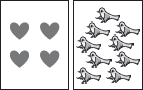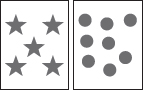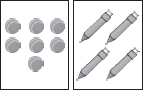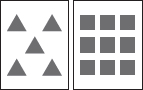# User ForumSubject :IMO    Class : Class 1

Which pair of cards has even sum?

ABCDA. 4 9 = 13, Odd B. 5 8 = 13, Odd C. 7 4 = 11, Odd D. 5 9 = 14, Even